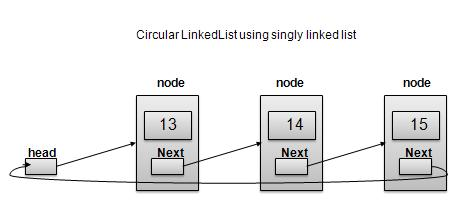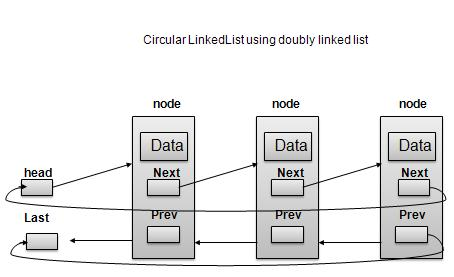# DSA using C - Circular Linked List

## Overview

Circular Linked List is a variation of Linked list in which first element points to last element and last element points to first element. Both Singly Linked List and Doubly Linked List can be made into as circular linked list.

## Singly Linked List as Circular## Doubly Linked List as CircularAs per above shown illustrations, following are the important points to be considered.

• Last Link'next points to first link of the list in both cases of singly as well as doubly linked list.

• First Link's prev points to the last of the list in case of doubly linked list.

## Basic Operations

Following are the important operations supported by a circular list.

• insert − insert an element in the start of the list.

• delete − insert an element from the start of the list.

• display − display the list.

## Length Operation

Following code demonstrate insertion operation at in a circular linked list based on single linked list.

```//insert link at the first location
void insertFirst(int key, int data){
struct node *link = (struct node*) malloc(sizeof(struct node));
if (isEmpty()) {
} else {
//point it to old first node

//point first to new first node
}
}
```

## Deletion Operation

Following code demonstrate deletion operation at in a circular linked list based on single linked list.

```//delete first item
struct node * deleteFirst(){
}
//mark next to first link as first

}
```

## Display List Operation

Following code demonstrate display list operation in a circular linked list.

```//display the list
void printList(){
printf("\n[ ");
//start from the beginning
while(ptr->next != ptr){
printf("(%d,%d) ",ptr->key,ptr->data);
ptr = ptr->next;
}
}
printf(" ]");
}
```

## Example

```#include <stdio.h>
#include <string.h>
#include <stdlib.h>
#include <stdbool.h>

struct node {
int data;
int key;
struct node *next;
};

struct node *current = NULL;

bool isEmpty(){
}
int length(){
int length = 0;

//if list is empty
return 0;
}
length++;
current = current->next;
}
return length;
}
//insert link at the first location
void insertFirst(int key, int data){
struct node *link = (struct node*) malloc(sizeof(struct node));
if (isEmpty()) {
} else {
//point it to old first node

//point first to new first node
}
}
//delete first item
struct node * deleteFirst(){
}
//mark next to first link as first

}
//display the list
void printList(){
printf("\n[ ");

//start from the beginning
while(ptr->next != ptr){
printf("(%d,%d) ",ptr->key,ptr->data);
ptr = ptr->next;
}
}
printf(" ]");
}
main() {
insertFirst(1,10);
insertFirst(2,20);
insertFirst(3,30);
insertFirst(4,1);
insertFirst(5,40);
insertFirst(6,56);
printf("Original List: ");

//print list
printList();

while(!isEmpty()){
struct node *temp = deleteFirst();
printf("\nDeleted value:");
printf("(%d,%d) ",temp->key,temp->data);
}
printf("\nList after deleting all items: ");
printList();
}
```

## Output

If we compile and run the above program then it would produce following output −

```Original List:
[ (6,56) (5,40) (4,1) (3,30) (2,20) ]
Deleted value:(6,56)
Deleted value:(5,40)
Deleted value:(4,1)
Deleted value:(3,30)
Deleted value:(2,20)
Deleted value:(1,10)
List after deleting all items:
[ ]
```